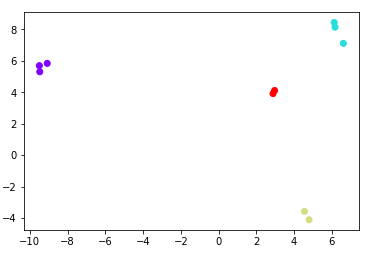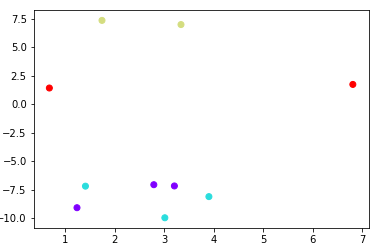# How to create synthetic datasets in Python?

So, how to create synthetic datasets in Python using the Scikit-learn library?

Real-world datasets are random, unorganized and require a lot of cleaning. Only then, it becomes useful for Data analysis and Machine learning purposes. In such a scenario, we can resort to creating our own synthetic datasets.

What is a synthetic dataset?

Synthetic datasets are artificial datasets. The values available in such datasets may be random or may follow specific statistical parameters such as standard deviation and mean.

We can easily create datasets with the required number of columns and characteristics. Let’s jump into the steps immediately.

We can create synthetic datasets by using make_blobs function in Python’s Scikit-Learn. Let’s see how.

## Importing make_blobs

make_blobs function creates synthetic datasets, hence we import the function directly from the Scikit-learn library.

```from sklearn.datasets import make_blobs
```

## make_blobs attributes

Now, there are 4 important attributes available in the make_blobs function.

1. n_samples
2. n_features
3. centers
4. cluster_std

n_samples – denotes the number of data points/rows in our datasets.

n_features – denotes the number of features/columns in our dataset

centers – denotes the number of categories in our dataset. That is one row may belong to category “1” and the other row may belong to category “2” or “3”.

cluster_std – denotes the Standard deviation of the clusters.

## To create synthetic datasets in Python

```artificial_data = make_blobs(n_samples=10)
```

Now, let’s check what is in store in the variable “artificial_data“.

```artificial_data
```
```(array([[ 1.69755924,  5.37195601],
[ 1.33002698,  7.97116204],
[-3.62757856, -5.85910081],
[ 2.50302847,  4.58667389],
[ 1.10209947,  7.96238836],
[ 0.0528797 ,  3.54315479],
[-3.24847154, -9.1346831 ],
[-4.38053923, -6.86488985],
[ 1.20784475,  5.5567213 ],
[-0.15842048,  8.86716747]]), array([0, 2, 1, 0, 2, 0, 1, 1, 0, 2]))```

Let’s check the type of the data above.

```type(artificial_data)
```
`tuple`

Just take a look at the above data, which is in the form of a tuple. You will observe that there are two Numpy arrays in it. Let’s look at the first Numpy array.

```artificial_data
```
```array([[ 1.69755924,  5.37195601],
[ 1.33002698,  7.97116204],
[-3.62757856, -5.85910081],
[ 2.50302847,  4.58667389],
[ 1.10209947,  7.96238836],
[ 0.0528797 ,  3.54315479],
[-3.24847154, -9.1346831 ],
[-4.38053923, -6.86488985],
[ 1.20784475,  5.5567213 ],
[-0.15842048,  8.86716747]])```

We will use the shape attribute to find the number of rows and columns in the above array.

```artificial_data.shape
```
`(10, 2)`

As we have included in the input, we have 10 rows and by default, it has 2 columns.

Now, we will view the second Numpy array in our dataset.

```artificial_data
```
`array([0, 2, 1, 0, 2, 0, 1, 1, 0, 2])`

The above array is the category to which each row belongs to. The first row belongs to the category “0”, second to the category “2” and so on.

### Visualization

To have a better understanding of the data, let’s visualize it on a scatter plot.

```import matplotlib.pyplot as plt
plt.scatter(artificial_data[:,0],artificial_data[:,1])
```## Controlling the number of columns

We can control the number of columns using the n_features attribute. For example, let’s increase the number of columns to 4.

```artificial_data = make_blobs(n_samples=10, n_features=4)
```
```(array([[  7.0540121 ,   9.40423006,  -5.20479579,  10.09114031],
[ -6.39325631,  -7.19221676,  -7.9021439 ,  -6.30629594],
[ -5.11591697, -11.60919477,  -8.2279402 ,  -7.38336542],
[  5.24573134,   6.751182  ,  -4.69953769,   8.69490957],
[ 10.19779164,   5.67052931,  -2.53900305,  -3.73241694],
[  9.90259193,   6.5701852 ,  -4.8635016 ,  -4.21959193],
[  4.60575269,  10.10264671,  -5.94821184,   8.1874132 ],
[  5.57674134,   9.51462632,  -6.02782592,  10.58370619],
[  8.78707389,   6.45824639,  -3.32942028,  -4.21336288],
[ -3.87442784, -10.16960876,  -7.44272906,  -6.42915496]]),
array([0, 1, 1, 0, 2, 2, 0, 0, 2, 1]))```

## Controlling the number of categories

If we want to increase the categories, then we can use the centers attribute.

Let’s increase the categories to 4. Also, reduce the columns to 2.

```artificial_data = make_blobs(n_samples=10, n_features=2,
centers=4)
```
```(array([[ 2.87227558,  5.69400793],
[ 4.77975749,  8.76058575],
[ 5.00783165,  6.48895964],
[-0.79027974,  6.85321449],
[ 7.17193582,  9.18745432],
[ 6.83707354,  8.08253888],
[ 9.36128373,  5.54509284],
[ 8.97269258,  5.89833823],
[-0.60840317,  7.62764434],
[ 4.83847334,  8.84703571]]),
array([3, 3, 0, 2, 1, 1, 0, 0, 2, 1]))```

Now, in the second array in the above result, we can find 4 categories namely 0,1,2 and 3.

### Visualization

```import matplotlib.pyplot as plt
plt.scatter(artificial_data[:,0],artificial_data[:,1],
c = artificial_data, cmap = 'rainbow')
```## Controlling the standard deviation of the cluster

Use the attribute cluster_std attribute to control the standard deviation of the data points from the cluster center.

### cluster_std is low

If the standard deviation is very low, then the points which belong to the same category group together in the scatter plot. This is because, since the standard deviation value is less, the spread of the points is less from the cluster center.

### Visualization

Let’s take the cluster_std to be 0.5.

```artificial_data = make_blobs(n_samples=10, n_features=2,
centers=4, cluster_std = 0.5)

import matplotlib.pyplot as plt
plt.scatter(artificial_data[:,0],artificial_data[:,1],
c = artificial_data, cmap = 'rainbow')
```### cluster_std is high

If the standard deviation is high, then the points which belong to same categories mix up with other categories.

### Visualization

Let’s take the cluster_std= 2.

```artificial_data = make_blobs(n_samples=10, n_features=2,
centers=4, cluster_std = 2)

import matplotlib.pyplot as plt
plt.scatter(artificial_data[:,0],artificial_data[:,1],
c = artificial_data, cmap = 'rainbow')
```## Conclusion

So, this is how you can create synthetic datasets with Python. Such datasets are very much useful for the K-Means algorithm implementation. Also for creating data science-related training and tutorials. If you have any doubts please post it in the comments.

If you found this post useful, do give it a thumbs up and share it among your friends.

Also, take a look at some of my other useful articles. Links below.

Learn screen automation the easy way

How to plot two histograms in a single plot?

See y’all!